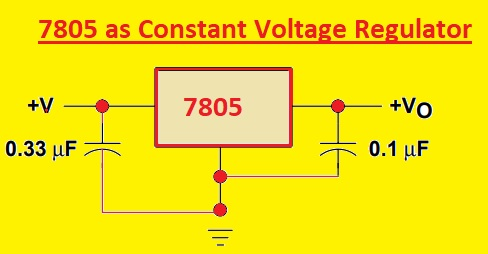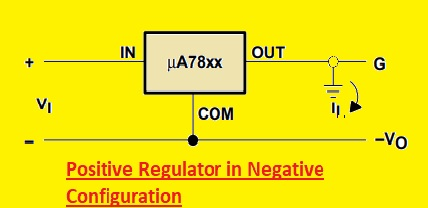Hello, readers welcome to the new post. Today we will discuss Introduction to 7805. Any type of voltage source is not able to provide a constant output since fluctuation exists in voltage source circuits. To get the constant voltage at the output there is a need for a voltage regulator that maintains the voltage value at the constant point. Integrated circuit used for voltage regulation that called a voltage regulator.

There are different types of voltage regulators used in electronic circuits according to applications that provide the constant voltage for projects like 7812, in this post we will discuss the 7805 IC. This IC belongs to the 78xx series of voltage regulators. That is fixed series linear voltage regulators and XX in this series denotes the value of voltage that certain ic provides. For example, 7805 is a +5V dc voltage regulator which means it will have five volts at the output irrespective of input voltages. In this tutorial, we will cover different parameters for 7805 to get an overview of this voltage regulator. So let’s get started  Introduction to 7805

### Introduction to 7805

• The 7805 IC is a voltage regulator that is the main part of small-size projects that need voltage up to five volts since it provides the plus five volts at the output.
• Voltage regulators have become necessary for electronic engineering and circuits and are the main part of circuits.
• The number 7805 is a combination of two numbers 78 which indicates it is a positive voltage regulator which means any voltage regulator that has 78 will provide positive voltage and 05 means output volts are five volts.
• This regulator has a 1.5 ampers output current. It causes heat losses so needs a heat sink during operation### 7805 Features

• The main features of this voltage regulator are explained here
• The common packaging that covers this regulator is TO-220 and KTE
• The temperature for this component is 125C
• It consists of inner thermal overload and short circuit current limiter circuits
• Its operating current is five milliamperes
• Its input voltage is seven to twenty-five volts
• Its input is plus five volts

### 7805 Pinout

• It has three pinouts that are listed here
• V+: That is the input voltage pinout
• GND is the ground terminal.
• Vo: is plus five volts output pin### 7805 IC Heating Issues

• This voltage regulator faces heat losses during operation since it has a 1.5A current value. It losses the high power. To minimize the power losses it needs a heatsink in circuits but there is proper measurement needed to use a heat sink for this component.
• Here is a formula that helps to find the heat generated by this regulator

Heat produced= (Input voltage-5) x O/P Current

• Let us suppose that we have 10 volts input volts and 0.3A current at the output
• So after putting values in the above equation we have
• (10-5)x0.3= 1.5W
• So these parameters there is 1.5W power will this regulator wasted in form of heat. So according to this power value, we will use a heat sink. But you have different values of input volts and the output current power wasted will be different so different values of heat sink will be used

### 7805 Voltage Regulator IC Schematic### 7805 as a Constant Voltage Regulator

• Here we will discuss the circuit that provides the plus five volts at the output and 7805 working as a constant voltage regulator.
• This circuit has a 33uF ceramic capacitor at the input side and a 0.1uF capacitor output side.
• Input capacitors solved the inductance issues of the circuit and the output current will remove the pulses from the current and make pure dc at the output side.
• At input which value of volts we provide will be converted into five volts at the output### 7805 as adjustable output Regulator

• This regulator also has features of variable output volts means with getting the constant volt we can get different values of volts according to circuit needs.
• Here you can see the variable output circuit that has two resistances R1  and R2 through varying the values of this resistance we can choose the output volts according to the input volts### LM7805 is a Linear or switching voltage regulator

• Voltage regulators are of two types linear and switching voltage regulators. LM7805 is the linear voltage regulator. Here are some points explained that will help you find the difference between linear and switching voltage regulations

Linear Voltage Regulators

• Regulators that use linear techniques for the regulation of voltage are called a linear regulators.
• Their design is buck circuits
• They have low to medium to high efficiency
• Their structure is less complicated
• Its prices are low
• Used to power low power using devices
• Common examples of linear regulators are LM7805, LM317

Switching Voltage Regulators

• These regulators regulate the voltage through switching circuits through on and off configuration
• Their designs are a buck, boost, and buck-boost
• They have high efficiency than linear regulators
• Their circuits are more complicated than linear
• They used in high-power projects
• A common example is LM3671

### 7805 as Positive Regulator in Negative Configuration

• The below circuit shows the Positive Regulator in a Negative Configuration of 7805### LM7805  Applications

• The main applications of LM7805 are explained here
• It used in reverse-biased protection circuits
• Used for output polarity reversal protection circuit
• Used in the circuit of dual supply of regulated
• Part of the current regulator’s circuits
• Used as an adjustable output regulator
• it provides the fix voltages
• Positive Regulator in Negative Configuration

That is all about the 7805 Voltage Regulator all details has explained. If you have any questions ask them here